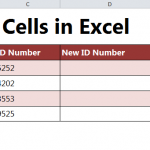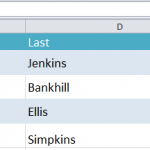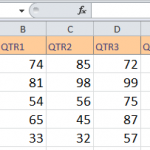# Category - Intermediate Excel Tutorials

## How to do an IF Statement in ExcelLearn how to do an IF Statement in Excel with our easy guide. An IF statement is when you enter a formula into Excel that …

## Combining Names in ExcelFollow this tutorial to learn about combining names in Excel, such as first and last names. The formula for putting two cells (that may contain …

## Tutorial: Prepend Text in ExcelFollow our Tutorial: Prepend Text in Excel to learn how to easily add text to existing information in new columns and rows. Learn the simple …

## Formula for Appending Text in ExcelLearn the formula for appending text in Excel with our guide. The act of appending text within an Excel spreadsheet means you are taking existing …

## Applying Conditional Formatting TutorialApplying conditional formatting to an Excel spreadsheet is great feature to know. The example in the tutorial shows how the Excel program can track birthdays …

## Excel Text Function TutorialExcel’s text functions can be used for charts, presentations, and general data organization. Follow the Excel Text Function Tutorial to learn how to utilize this …

## Chart Creation in ExcelMultiple types of charts can be easily created using Excel. Chart creation in Excel includes bar graphs, pie graphs, and many more. Using the tutorial …

## How to Sort and Filter in ExcelWith Excel, you can sort and filter through any amount of data. These functions can help to find data patterns, display specific information, or create …

## How to Create Pivot Tables in ExcelCreating a pivot table in Excel allows the user to quickly and easily summarize and arrange large amounts of data, and then pull certain details …

## Calculating Averages in Excel TutorialAlong with basic math formulas, Excel also has the ability to calculate averages. Since solving averages by hand is boring and tedious, let a computer …

Page 2 of 2